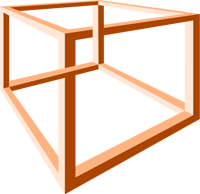# Algebra 2

•Algebra 2

District description of the class:

Algebra 2 is the capstone course of the three units of credit required for a Regents diploma.  This course is a continuation and extension of the two courses that preceded it.

While developing the algebraic techniques that will be required of those students that continue their study of mathematics, this course is also intended to continue developing alternative solution strategies and algorithms. For example, technology can provide to many students the means to address a problem situation to which they might not otherwise have access.

Within this course, the number system will be extended to include imaginary and complex numbers.  The families of functions to be studied will include polynomial, absolute value, radical, trigonometric, exponential, and logarithmic functions. Problem situations involving direct and indirect variation will be solved. Problems resulting in systems of equations will be solved graphically and algebraically. Algebraic techniques will be developed to facilitate rewriting mathematical expressions into multiple equivalent forms.

Data analysis will be extended to include measures of dispersion and the analysis of regression that model functions studied throughout this course. Associated correlation coefficients will be determined, using technology tools and interpreted as a measure of strength of the relationship.

Arithmetic and geometric sequences will be expressed in multiple forms, and arithmetic and geometric series will be evaluated. Binomial experiments will provide the basis for the study of probability theory and the normal probability distribution will be analyzed and used as an approximation for these binomial experiments.

Important Sites and resources to be successful

EXTRA MATH RESOURCES

Pearson Success Net  log-in for lessons and student edition of the textbook

Oswego City School District Regents Review - provides lessons and self graded quizzes

# Related Files

•# Formula Sheet and Study Guide

•